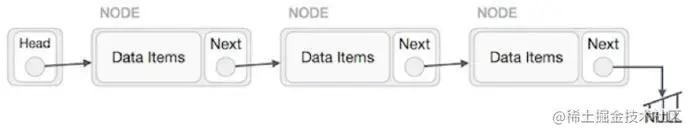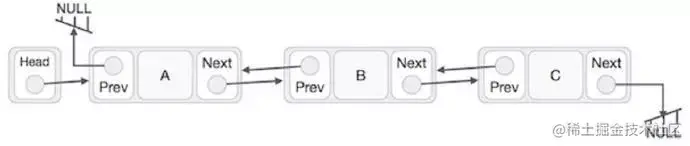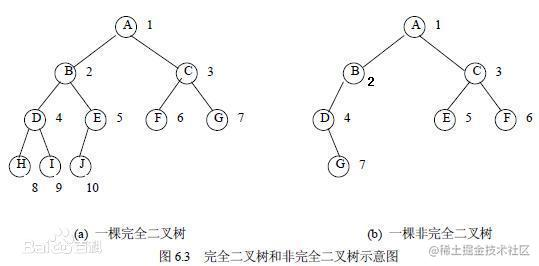# iOS开发总结(一)数据结构和算法

## 数据结构

1. 数据结构的存储一般常用的有几种？各有什么特点？
顺序存储结构:
比如，数组，1-2-3-4-5-6-7-8-9-10，存储是按顺序的。再比如栈和队列等

链式存储结构:
比如，数组，1-2-3-4-5-6-7-8-9-10，链式存储就不一样了 1(地址)-2(地址)-7(地址)-4(地址)-5(地址)-9(地址)-8(地址)-3(地址)-6(地址)-10(地址)。每个数字后面跟着一个地址 而且存储形式不再是顺序

• 集合结构

一个集合，就是一个圆圈中有很多个元素，元素与元素之间没有任何关系 这个很简单

• 线性结构

一个条线上站着很多个人。 这条线不一定是直的。也可以是弯的。也可以是直的 相当于一条线被分成了好几段的样子 （发挥你的想象力）。 线性结构是一对一的关系

• 树形结构

做开发的肯定或多或少的知道xml 解析 树形结构跟他非常类似。也可以想象成一个金字塔。树形结构是一对多的关系

• 图形结构

这个就比较复杂了。他呢 无穷。无边 无向（没有方向）图形机构 你可以理解为多对多 类似于我们人的交集关系

• 单向链表 A->B->C->D->E->F->G->H. 这就是单向链表 H 是头 A 是尾 像一个只有一个头的火车一样 只能一个头拉着跑• 双向链表• 循环链表

循环链表是与单向链表一样，是一种链式的存储结构，所不同的是，循环链表的最后一个结点的指针是指向该循环链表的第一个结点或者表头结点，从而构成一个环形的链。发挥想象力 A->B->C->D->E->F->G->H->A. 绕成一个圈。就像蛇吃自己的这就是循环 不需要去死记硬背哪些理论知识。

• 数组

数组元素在内存上连续存放，可以通过下标查找元素；插入、删除需要移动大量元素，比较适用于元素很少变化的情况

• 链表

链表中的元素在内存中不是顺序存储的，查找慢，插入、删除只需要对元素指针重新赋值，效率高

2. #### 堆

• 堆是一种经过排序的树形数据结构，每个节点都有一个值，通常我们所说的堆的数据结构是指二叉树。所以堆在数据结构中通常可以被看做是一棵树的数组对象。而且堆需要满足一下两个性质：

1）堆中某个节点的值总是不大于或不小于其父节点的值

2）堆总是一棵完全二叉树。(完全二叉树是效率很高的数据结构，完全二叉树是由满二叉树而引出来的。对于深度为K的，有n个结点的二叉树，当且仅当其每一个结点都与深度为K的满二叉树中编号从1至n的结点一一对应时称之为完全二叉树。

•• 堆分为两种情况，有最大堆和最小堆。将根节点最大的堆叫做最大堆或大根堆，根节点最小的堆叫做最小堆或小根堆，在一个摆放好元素的最小堆中，父结点中的元素一定比子结点的元素要小，但对于左右结点的大小则没有规定谁大谁小。

• 堆常用来实现优先队列，堆的存取是随意的，这就如同我们在图书馆的书架上取书，虽然书的摆放是有顺序的，但是我们想取任意一本时不必像栈一样，先取出前面所有的书，书架这种机制不同于箱子，我们可以直接取出我们想要的书。

#### 栈

• 栈是限定仅在表尾进行插入和删除操作的线性表。我们把允许插入和删除的一端称为栈顶，另一端称为栈底，不含任何数据元素的栈称为空栈。栈的特殊之处在于它限制了这个线性表的插入和删除位置，它始终只在栈顶进行。

• 栈是一种具有后进先出的数据结构，又称为后进先出的线性表，简称 LIFO（Last In First Out）结构。也就是说后存放的先取，先存放的后取，这就类似于我们要在取放在箱子底部的东西（放进去比较早的物体），我们首先要移开压在它上面的物体（放进去比较晚的物体）。

• 堆栈中定义了一些操作。两个最重要的是PUSH和POP。PUSH操作在堆栈的顶部加入一个元素。POP操作相反，在堆栈顶部移去一个元素，并将堆栈的大小减一。

• 栈的应用—递归

#### 队列

• 队列是只允许在一端进行插入操作、而在另一端进行删除操作的线性表。允许插入的一端称为队尾，允许删除的一端称为队头。它是一种特殊的线性表，特殊之处在于它只允许在表的前端进行删除操作，而在表的后端进行插入操作，和栈一样，队列是一种操作受限制的线性表。

• 队列是一种先进先出的数据结构，又称为先进先出的线性表，简称 FIFO（First In First Out）结构。也就是说先放的先取，后放的后取，就如同行李过安检的时候，先放进去的行李在另一端总是先出来，后放入的行李会在最后面出来。

3. ###### 二叉树的结点定义如下：
``````struct BinaryTreeNode
{
int m_nValue ；
BinaryTreeNode* m_pLeft;
BinarvTreeNode* m_pRight ；
}
复制代码``````
• 如果一棵树只有一个结点，它的深度为1。

• 如果根结点只有左子树而没有右子树，那么树的深度应该是其左子树的深度加1；同样，如果根结点只有右子树而没有左子树，那么树的深度应该是其右子树的深度加1。

• 如果既有右子树又有左子树，那该树的深度就是其左、右子树深度的较大值再加1。

``````int TreeDepth(TreeNode* pRoot)
{
if(pRoot == nullptr)
return 0;
int left = TreeDepth(pRoot->left);
int right = TreeDepth(pRoot->right);

return (left>right) ? (left+1) : (right+1);
}
复制代码``````

4. 输入一棵二叉树的根结点，判断该树是不是平衡二叉树？

• 重复遍历结点

• 遍历一遍结点

##### 方法1:
``````struct TreeNode{
int val;
TreeNode* left;
TreeNode* right;
};

int TreeDepth(TreeNode* pRoot){
if(pRoot==NULL)
return 0;
int left=TreeDepth(pRoot->left);
int right=TreeDepth(pRoot->right);
return left>right?(left+1):(right+1);
}

bool IsBalanced(TreeNode* pRoot){
if(pRoot==NULL)
return true;
int left=TreeDepth(pRoot->left);
int right=TreeDepth(pRoot->right);
int diff=left-right;
if(diff>1 || diff<-1)
return false;
return IsBalanced(pRoot->left) && IsBalanced(pRoot->right);
}

##### 方法2：
``````bool IsBalanced_1(TreeNode* pRoot,int& depth){
if(pRoot==NULL){
depth=0;
return true;
}
int left,right;
int diff;
if(IsBalanced_1(pRoot->left,left) && IsBalanced_1(pRoot->right,right)){
diff=left-right;
if(diff<=1 || diff>=-1){
depth=left>right?left+1:right+1;
return true;
}
}
return false;
}

bool IsBalancedTree(TreeNode* pRoot){
int depth=0;
return IsBalanced_1(pRoot,depth);
}

#### 算法

• 时间频度

一个算法执行所耗费的时间,从理论上是不能算出来的,必须上机运行测试才能知道.但我们不可能也没有必要对每个算法都上机测试,只需知道哪个算法花费的时间多,哪个算法花费的时间少就可以了.并且一个算法花费的时间与算法中语句的执行次数成正比例,哪个算法中语句执行次数多,它花费时间就多.一个算法中的语句执行次数称为语句频度或时间频度.记为T(n).

• 时间复杂度

一般情况下,算法中基本操作重复执行的次数是问题规模n的某个函数,用T(n)表示,若有某个辅助函数f(n),使得当n趋近于无穷大时,T（n)/f(n)的极限值为不等于零的常数,则称f(n)是T(n)的同数量级函数.记作T(n)=O(f(n)),称O(f(n)) 为算法的渐进时间复杂度,简称时间复杂度.

• 在各种不同算法中,若算法中语句执行次数为一个常数,则时间复杂度为O(1),另外,在时间频度不相同时,时间复杂度有可能相同,如T(n)=n2+3n+4与T(n)=4n2+2n+1它们的频度不同,但时间复杂度相同,都为O(n2).

• 按数量级递增排列,常见的时间复杂度有：

O(1)称为常量级，算法的时间复杂度是一个常数。

O(n)称为线性级，时间复杂度是数据量n的线性函数。

O(n²)称为平方级，与数据量n的二次多项式函数属于同一数量级。

O(n³)称为立方级，是n的三次多项式函数。

O(logn)称为对数级，是n的对数函数。

O(nlogn)称为介于线性级和平方级之间的一种数量级

O(2ⁿ)称为指数级，与数据量n的指数函数是一个数量级。

O(n!)称为阶乘级，与数据量n的阶乘是一个数量级。

它们之间的关系是： O(1)<O(logn)<O(n)<O(nlogn)<O(n²)<O(n³)<O(2ⁿ)<O(n!)，随着问题规模n的不断增大,上述时间复杂度不断增大,算法的执行效率越低.

#### 2.空间复杂度

• 评估执行程序所需的存储空间。可以估算出程序对计算机内存的使用程度。不包括算法程序代码和所处理的数据本身所占空间部分。通常用所使用额外空间的字节数表示。其算法比较简单，记为S(n)=O(f(n))，其中，n表示问题规模。

• ### 选择排序、冒泡排序、插入排序三种排序算法可以总结为如下：

都将数组分为已排序部分和未排序部分。

选择排序将已排序部分定义在左端，然后选择未排序部分的最小元素和未排序部分的第一个元素交换。

冒泡排序将已排序部分定义在右端，在遍历未排序部分的过程执行交换，将最大元素交换到最右端。

插入排序将已排序部分定义在左端，将未排序部分元的第一个元素插入到已排序部分合适的位置。

``````/**
*  【选择排序】：最值出现在起始端
*
*  第1趟：在n个数中找到最小(大)数与第一个数交换位置
*  第2趟：在剩下n-1个数中找到最小(大)数与第二个数交换位置
*  重复这样的操作...依次与第三个、第四个...数交换位置
*  第n-1趟，最终可实现数据的升序（降序）排列。
*
*/
void selectSort(int *arr, int length) {
for (int i = 0; i < length - 1; i++) { //趟数
for (int j = i + 1; j < length; j++) { //比较次数
if (arr[i] > arr[j]) {
int temp = arr[i];
arr[i] = arr[j];
arr[j] = temp;
}
}
}
}

/**
*  【冒泡排序】：相邻元素两两比较，比较完一趟，最值出现在末尾
*  第1趟：依次比较相邻的两个数，不断交换（小数放前，大数放后）逐个推进，最值最后出现在第n个元素位置
*  第2趟：依次比较相邻的两个数，不断交换（小数放前，大数放后）逐个推进，最值最后出现在第n-1个元素位置
*   ……   ……
*  第n-1趟：依次比较相邻的两个数，不断交换（小数放前，大数放后）逐个推进，最值最后出现在第2个元素位置
*/
void bublleSort(int *arr, int length) {
for(int i = 0; i < length - 1; i++) { //趟数
for(int j = 0; j < length - i - 1; j++) { //比较次数
if(arr[j] > arr[j+1]) {
int temp = arr[j];
arr[j] = arr[j+1];
arr[j+1] = temp;
}
}
}
}

/**
*  折半查找：优化查找时间（不用遍历全部数据）
*
*  折半查找的原理：
*   1> 数组必须是有序的
*   2> 必须已知min和max（知道范围）
*   3> 动态计算mid的值，取出mid对应的值进行比较
*   4> 如果mid对应的值大于要查找的值，那么max要变小为mid-1
*   5> 如果mid对应的值小于要查找的值，那么min要变大为mid+1
*
*/

// 已知一个有序数组, 和一个key, 要求从数组中找到key对应的索引位置
int findKey(int *arr, int length, int key) {
int min = 0, max = length - 1, mid;
while (min <= max) {
mid = (min + max) / 2; //计算中间值
if (key > arr[mid]) {
min = mid + 1;
} else if (key < arr[mid]) {
max = mid - 1;
} else {
return mid;
}
}
return -1;
}
复制代码``````

1. ``````void char_reverse (char *cha) {

// 定义头部指针
char *begin = cha;
// 定义尾部指针
char *end = cha + strlen(cha) -1;

while (begin < end) {

char temp = *begin;
*(begin++) = *end;//*crash
*(end--) = temp;
}
}复制代码``````
2. 链表反转（头插法）

• #### 实现

``````#import "ReverseList.h"
// 定义一个链表
struct Node {
int data;
struct Node *next;
};
// 链表反转

// 构造一个链表
struct Node* constructList(void);

// 打印链表中的数据

{
// 定义遍历指针，初始化为头结点

// 反转后的链表头部
struct Node *newH = NULL;

// 遍历链表
while (p != NULL) {

// 记录下一个结点
struct Node *temp = p->next;
// 当前结点的next指向新链表头部
p->next = newH;
// 更改新链表头部为当前结点
newH = p;
// 移动p指针
p = temp;
}

// 返回反转后的链表头结点
return newH;
}

struct Node* constructList(void)
{
// 头结点定义
// 记录当前尾结点
struct Node *cur = NULL;

for (int i = 1; i < 5; i++) {
struct Node *node = malloc(sizeof(struct Node));
node->data = i;

// 头结点为空，新结点即为头结点
}
// 当前结点的next为新结点
else{
cur->next = node;
}

// 设置当前结点为新结点
cur = node;
}

}

{
while (temp != NULL) {
printf("node is %d \n", temp->data);
temp = temp->next;
}
}

@end
复制代码``````

3. 如何查找第一个只出现一次的字符（Hash查找）

``````char findFirstChar(char* cha)
{
char result = '\0';

// 定义一个数组 用来存储各个字母出现次数
int array;

// 对数组进行初始化操作
for (int i=0; i<256; i++) {
array[i] =0;
}
// 定义一个指针 指向当前字符串头部
char* p = cha;
// 遍历每个字符
while (*p != '\0') {
// 在字母对应存储位置 进行出现次数+1操作
array[*(p++)]++;
}

// 将P指针重新指向字符串头部
p = cha;
// 遍历每个字母的出现次数
while (*p != '\0') {
// 遇到第一个出现次数为1的字符，打印结果
if (array[*p] == 1)
{
result = *p;
break;
}
// 反之继续向后遍历
p++;
}

return result;
}
复制代码``````
4. ``````- (NSArray <UIView *> *)findCommonSuperView:(UIView *)viewOne
other:(UIView *)viewOther
{
NSMutableArray *result = [NSMutableArray array];

// 查找第一个视图的所有父视图
NSArray *arrayOne = [self findSuperViews:viewOne];
// 查找第二个视图的所有父视图
NSArray *arrayOther = [self findSuperViews:viewOther];

int i = 0;
// 越界限制条件
while (i < MIN((int)arrayOne.count, (int)arrayOther.count)) {
// 倒序方式获取各个视图的父视图
UIView *superOne = [arrayOne objectAtIndex:arrayOne.count - i - 1];
UIView *superOther = [arrayOther objectAtIndex:arrayOther.count - i - 1];

// 比较如果相等 则为共同父视图
if (superOne == superOther) {
i++;
}
// 如果不相等，则结束遍历
else{
break;
}
}

return result;
}

- (NSArray <UIView *> *)findSuperViews:(UIView *)view
{
// 初始化为第一父视图
UIView *temp = view.superview;
// 保存结果的数组
NSMutableArray *result = [NSMutableArray array];
while (temp) {
// 顺着superview指针一直向上查找
temp = temp.superview;
}
return result;
}

复制代码``````

1. 中位数：是指一组数据从小到大排列，位于中间的那个数。数据个数为奇数时 中间的一个，数据个数为偶数时是2个的平均

``````//求一个无序数组的中位数
int findMedian(int a[], int aLen)
{
int low = 0;
int high = aLen - 1;

int mid = (aLen - 1) / 2;
int div = PartSort(a, low, high);

while (div != mid)
{
if (mid < div)
{
//左半区间找
div = PartSort(a, low, div - 1);
}
else
{
//右半区间找
div = PartSort(a, div + 1, high);
}
}
//找到了
return a[mid];
}

int PartSort(int a[], int start, int end)
{
int low = start;
int high = end;

//选取关键字
int key = a[end];

while (low < high)
{
//左边找比key大的值
while (low < high && a[low] <= key)
{
++low;
}

//右边找比key小的值
while (low < high && a[high] >= key)
{
--high;
}

if (low < high)
{
//找到之后交换左右的值
int temp = a[low];
a[low] = a[high];
a[high] = temp;
}
}

int temp = a[high];
a[high] = a[end];
a[end] = temp;

return low;
}
复制代码``````

5. 如何给定一个整数数组和一个目标值，找出数组中和为目标值的两个数。
思路一、穷举所有可能的两数相加，即遍历两次，直到找到为止，这种算法的时间复杂度为O(n2)O(n^2)O(n 2 )，空间复杂度为O(1) 。能实现，但不是最优方案

``````- (BOOL)twoNumSumWithTarget:(int)target Array:(NSArray<NSNumber *> *)array {

NSMutableArray *finalArray = [NSMutableArray array];

for (int i = 0; i < array.count; i++) {

for (int j = i + 1; j < array.count; j++) {

if ([array[i] intValue] + [array[j] intValue] == target) {